Classical Mechanics

# 2D Dynamics: Level 1-2 Challenges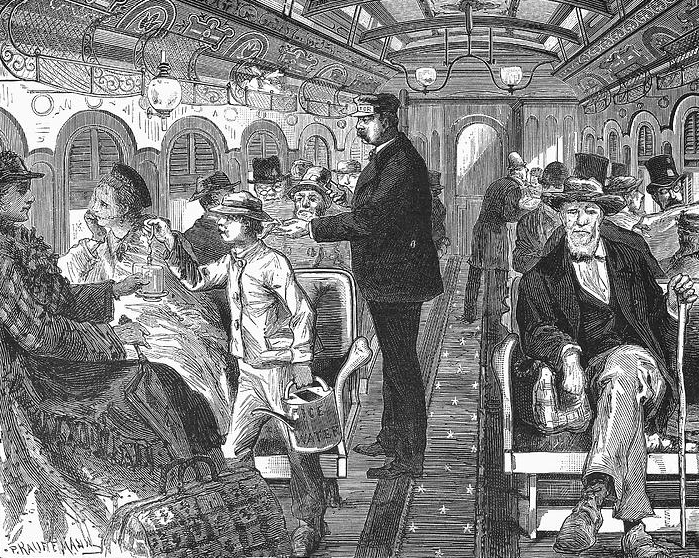On a moving train, a passenger who is seated facing the front, tosses a coin up in the air. Given that the coin falls into the lap of the person sitting behind this person, what can we say about the motion of the train?

Note:

• All the people are facing the front of the train.

• All the windows/doors are shut.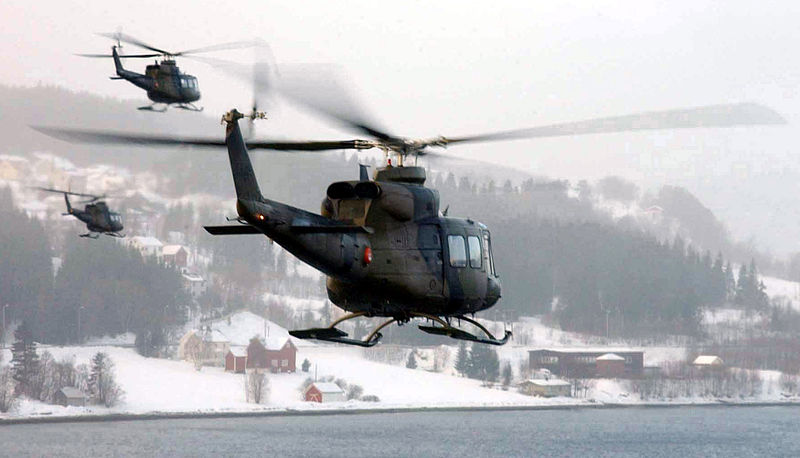You are hovering in a helicopter well above the Earth's surface such that the helicopter is suspended in mid air, stationary with respect to the Earth. The Earth is rotating at the same time. Why should you fly to my destination, if it will come to you due to Earth's rotation?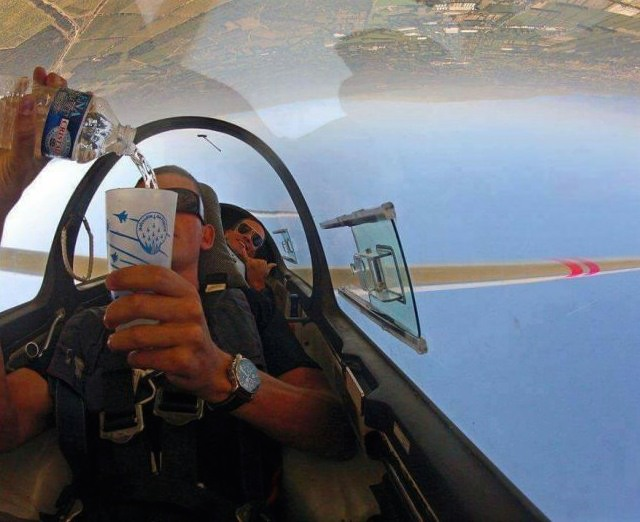As shown in the picture, water flows from the bottle into the cup. What explains this phenomena?

Details

• Note, the picture is upside down. The Earth is in the top of the photo and the bottom of the plane is facing the atmosphere.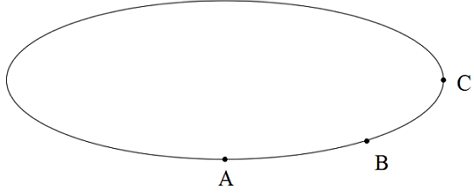A particle is moving on an elliptical path as shown, at a constant speed. At which point is the particle's acceleration at maximum?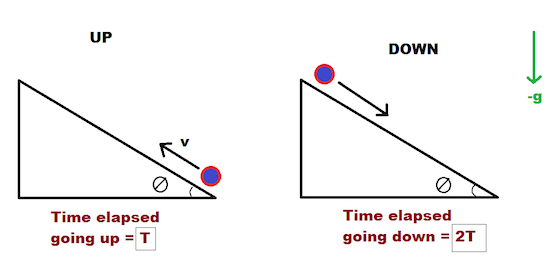A point-mass (particle) is shot up an inclined plane that makes an angle $$\theta$$ with the ground. It slides up the incline to a certain distance, and then slides all the way back down. However, due to friction, the time it takes for the particle to slide from the maximum distance it reaches up the incline back to the bottom is twice the time it takes for it to go to the maximum distance up the incline from the bottom.

The coefficient of friction $$\mu$$ between the particle and the incline can be written as $$\frac{a}{b}\tan\theta$$, where $$a$$ and $$b$$ are co-prime positive integers.

What is the value of $$a + b$$?

×